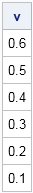A recent question posted on a discussion forum discussed storing the strictly upper-triangular portion of a correlation matrix. Suppose that you have a correlation matrix like the following:

```proc iml; corr = {1.0 0.6 0.5 0.4, 0.6 1.0 0.3 0.2, 0.5 0.3 1.0 0.1, 0.4 0.2 0.1 1.0};```

Every correlation matrix is symmetric and has a unit diagonal. Consequently, although this 4 x 4 matrix has 16 elements, only six elements convey any information. In general, an n x n matrix has only n(n–1)/2 informative elements. It seems logical, therefore, that for large matrices you might want to store only the strictly upper portion of a correlation matrix.

If the correlation matrix is stored in a data set, you can use the DATA step and arrays to extract only the strictly upper-triangular correlations. In the SAS/IML language, you can use the ROW and COL functions to extract the upper triangular portion of the matrix into a vector, as follows:

```r = row(corr); c = col(corr); upperTri = loc(r < c); /* upper tri indices in row major order */ v = corr[upperTri]; /* vector contains n*(n-1)/2 upper triangular corr */ print v;```To reconstruct the correlation matrix from the vector is a little challenging. The main problem is to figure out the dimension of the correlation matrix by using the number of elements in the vector v.

Let k be number of elements in the vector v. Then k = n(n–1)/2 elements for some value of n. Rearranging the equation gives n2 - n - 2k = 0, and by the quadratic formula this equation has the positive solution n = (1 + sqrt(1 + 8k) ) / 2. For example, k=6 for the present example, from which we deduce that n = 4.

After you have discovered the value of n, it is easy allocate a matrix, copy the correlations into the upper triangular portion, make the matrix symmetric, and assign the unit diagonal, as follows:

```k = nrow(v); n = (sqrt(1 + 8*k) + 1)/2; /* dimension of full matrix */ A = J(n,n,0); /* allocate zero matrix */ A[upperTri] = v; /* copy correlations */ A = A + A`; /* make symmetric */ A[loc(r = c)] = 1; /* put 1 on diagonal */```

If you use this operation frequently, you can create modules that encapsulate the process of extracting and restoring correlation matrices.

Do you like to solve tricky little problems? Do you enjoy spending a few minutes each day learning about SAS software and sharing your expertise with other? If so, you might enjoy participating in the SAS Support Communities. If you have written a paper about how to do something non-trivial in SAS, consider posting it to the SAS/IML File Exchange.

ShareDistinguished Researcher in Computational Statistics

Rick Wicklin, PhD, is a distinguished researcher in computational statistics at SAS and is a principal developer of SAS/IML software. His areas of expertise include computational statistics, simulation, statistical graphics, and modern methods in statistical data analysis. Rick is author of the books Statistical Programming with SAS/IML Software and Simulating Data with SAS.

1.Rick,
Here is another solution. No need to judge the dimemsion of Matrix .

proc iml;
v={0.6 0.5 0.4 0.3 0.2 0.1};

step=0;
d=0;
n=ncol(v)+1;
do while(n>step);
v=insert(v,{1},0,n-step);
d=d+1;
step=step+d;
end;
corr=sqrvech(v);
print corr;
quit;

•Yes. I prefer to avoid using the INSERT function inside a loop. For large matrices, the INSERT method results in a lot of allocating and copying.

2.Rick,
Here is another way to calculate the dimension of Matrix .

proc iml;
v={0.6 0.5 0.4 0.3 0.2 0.1 };

d=nrow(sqrvech(v));
corr=I(d+1);
corr[loc(row(corr)<col(corr))]=v;
corr=corr+corr`-I(d+1);
print corr;
qui

3.One of many useful tips I've learned from this blog: As shown a few years ago, if you're willing to extract the diagonal elements, things get really simple. sqrvech also lets you create a complete square correlation matrix A by entering only the lower triangle V, including the 1's on the diagonal.

*http://blogs.sas.com/content/iml/2012/03/21/creating-symmetric-matrices-two-useful-functions-with-strange-names.html;
proc iml;
corr = {1.0 0.6 0.5 0.4,
0.6 1.0 0.3 0.2,
0.5 0.3 1.0 0.1,
0.4 0.2 0.1 1.0};

*extract the lower triangle;
v = vech(corr);
print v;

*reconstruct the original;
a=sqrvech(v);
print a;